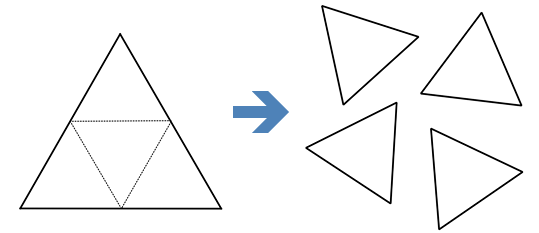# All I Care About Is The Perimeter

Geometry Level 1If I cut an equilateral triangle with a perimeter of 6 to 4 equal size equilateral triangles, what is the total perimeter of all these 4 smaller triangles?

×